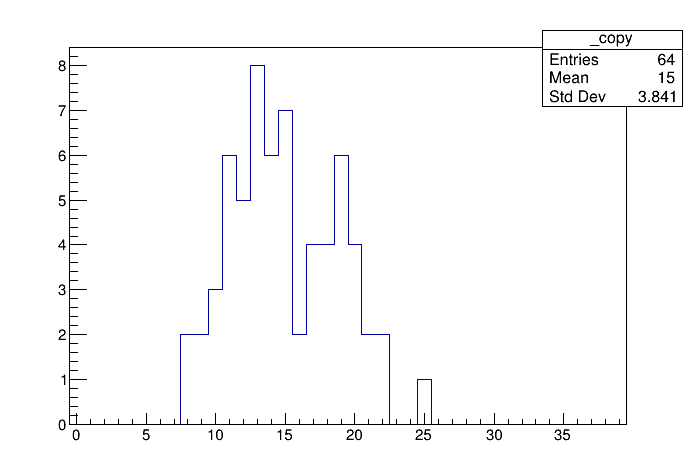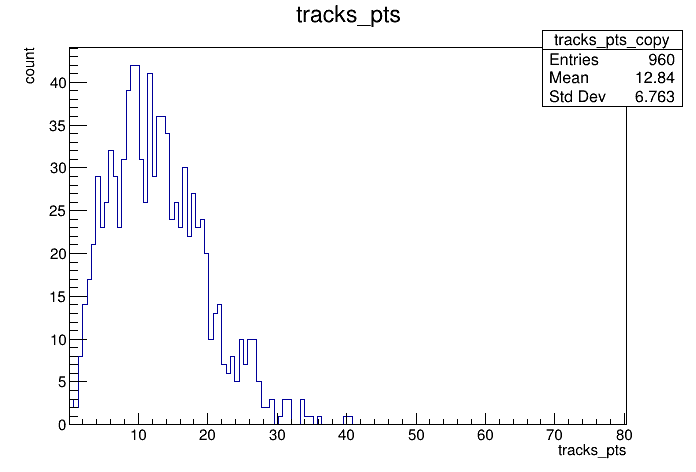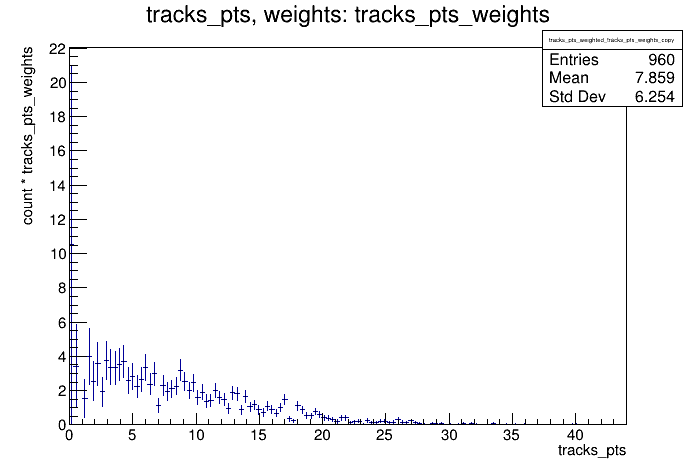# Df 0 0 2_Data Model¶

Show how to work with non-flat data models, e.g. vectors of tracks.

This tutorial shows the possibility to use data models which are more complex than flat ntuples with RDataFrame

Author: Danilo Piparo (CERN)
This notebook tutorial was automatically generated with ROOTBOOK-izer from the macro found in the ROOT repository on Tuesday, June 15, 2021 at 07:13 AM.

In :
using FourVector = ROOT::Math::XYZTVector;
using FourVectorVec = std::vector<FourVector>;
using FourVectorRVec = ROOT::VecOps::RVec<FourVector>;
using CylFourVector = ROOT::Math::RhoEtaPhiVector;


A simple helper function to fill a test tree: this makes the example stand-alone.

In :
%%cpp -d
void fill_tree(const char *filename, const char *treeName)
{
const double M = 0.13957; // set pi+ mass
TRandom3 R(1);

auto genTracks = [&](){
FourVectorVec tracks;
const auto nPart = R.Poisson(15);
tracks.reserve(nPart);
for (int j = 0; j < nPart; ++j) {
const auto px = R.Gaus(0, 10);
const auto py = R.Gaus(0, 10);
const auto pt = sqrt(px * px + py * py);
const auto eta = R.Uniform(-3, 3);
const auto phi = R.Uniform(0.0, 2 * TMath::Pi());
CylFourVector vcyl(pt, eta, phi);
// set energy
auto E = sqrt(vcyl.R() * vcyl.R() + M * M);
// fill track vector
tracks.emplace_back(vcyl.X(), vcyl.Y(), vcyl.Z(), E);
}
return tracks;
};

ROOT::RDataFrame d(64);
d.Define("tracks", genTracks).Snapshot<FourVectorVec>(treeName, filename, {"tracks"});
}


We prepare an input tree to run on

In :
auto fileName = "df002_dataModel.root";
auto treeName = "myTree";
fill_tree(fileName, treeName);


We read the tree from the file and create a rdataframe, a class that allows us to interact with the data contained in the tree.

In :
ROOT::RDataFrame d(treeName, fileName, {"tracks"});


## Operating on branches which are collection of objects¶

Here we deal with the simplest of the cuts: we decide to accept the event only if the number of tracks is greater than 5.

In :
auto n_cut = [](const FourVectorRVec &tracks) { return tracks.size() > 8; };
auto nentries = d.Filter(n_cut, {"tracks"}).Count();

std::cout << *nentries << " passed all filters" << std::endl;

62 passed all filters


Another possibility consists in creating a new column containing the quantity we are interested in. In this example, we will cut on the number of tracks and plot their transverse momentum.

In :
auto getPt = [](const FourVectorRVec &tracks) {
return ROOT::VecOps::Map(tracks, [](const FourVector& v){return v.Pt();});
};


We do the same for the weights.

In :
auto getPtWeights = [](const FourVectorRVec &tracks) {
return ROOT::VecOps::Map(tracks, [](const FourVector& v){ return 1. / v.Pt();});
};

auto augmented_d = d.Define("tracks_n", [](const FourVectorRVec &tracks) { return (int)tracks.size(); })
.Filter([](int tracks_n) { return tracks_n > 2; }, {"tracks_n"})
.Define("tracks_pts", getPt)
.Define("tracks_pts_weights", getPtWeights);

auto trN = augmented_d.Histo1D({"", "", 40, -.5, 39.5}, "tracks_n");
auto trPts = augmented_d.Histo1D("tracks_pts");
auto trWPts = augmented_d.Histo1D("tracks_pts", "tracks_pts_weights");

auto c1 = new TCanvas();
trN->DrawCopy();

auto c2 = new TCanvas();
trPts->DrawCopy();

auto c3 = new TCanvas();
trWPts->DrawCopy();

return 0;


Draw all canvases

In :
gROOT->GetListOfCanvases()->Draw()